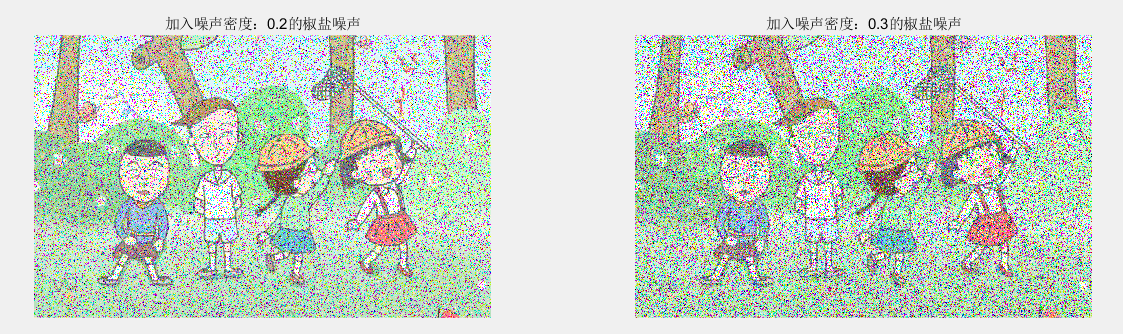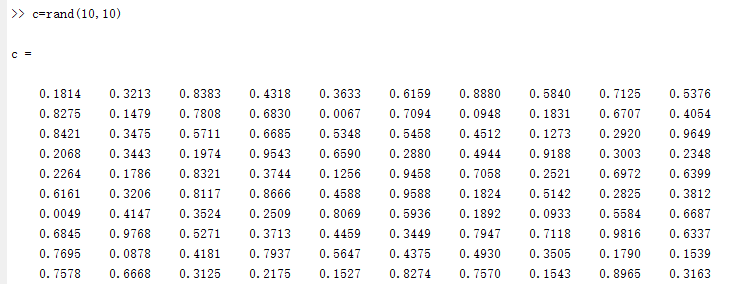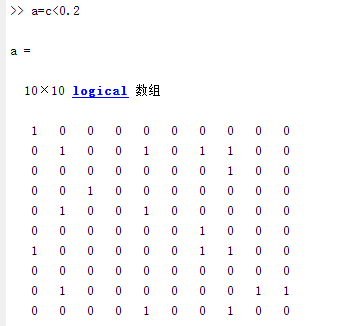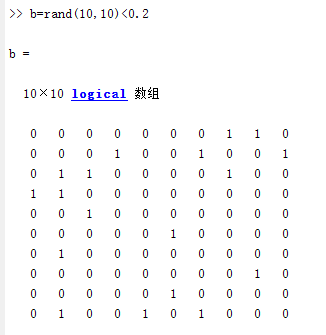2017-12-15 11:01:55 vitaminc4 阅读数 1161

### 椒盐噪声：

2019-09-16 20:05:16 weixin_44225182 阅读数 2363

椒盐噪声也称为脉冲噪声，是图像中经常见到的一种噪声，它是一种随机出现的白点或者黑点，可能是亮的区域有黑色像素或是在暗的区域有白色像素（或是两者皆有）。盐和胡椒噪声的成因可能是影像讯号受到突如其来的强烈干扰而产生、类比数位转换器或位元传输错误等。例如失效的感应器导致像素值为最小值，饱和的感应器导致像素值为最大值。

``````t=imread('a1.jpg');
subplot(1,2,1),imshow(t),title('原图');
t1=imnoise(t,'salt & pepper',0.1);
subplot(1,2,2),imshow(t1),title('加入噪声密度：0.1的椒盐噪声');
t2=imnoise(t,'salt & pepper',0.2);
figure,subplot(1,2,1),imshow(t2),title('加入噪声密度：0.2的椒盐噪声');
t3=imnoise(t,'salt & pepper',0.3);
subplot(1,2,2),imshow(t3),title('加入噪声密度：0.3的椒盐噪声');
``````• 噪声类型是 ‘salt & pepper’ 一定注意空格的位置 这里比较严格
• 当噪声类型是’salt & pepper’的时候，第三个参数的意思是噪声密度，比如0.1，那么总像素个数的10%为黑白点，当然是黑点还是白点都是随机的。

``````image=imread('a1.jpg');
[width,height,z]=size(image);

result2=image;
subplot(1,2,1)
imshow(image);
title('原图');

%k1、k2作为判断临界点
k1=0.2;
k2=0.2;
%rand(m,n)是随机生成m行n列的矩阵，每个矩阵元素都在0-1之间
%这里k都是0.2，所以小于k的元素在矩阵中为1，反之为0
a1=rand(width,height)<k1;
a2=rand(width,height)<k2;
%合成彩色图像
t1=result2(:,:,1);
t2=result2(:,:,2);
t3=result2(:,:,3);
%分成黑点 白点 随机
t1(a1&a2)=0;
t2(a1&a2)=0;
t3(a1&a2)=0;
t1(a1& ~a2)=255;
t2(a1& ~a2)=255;
t3(a1& ~a2)=255;
result2(:,:,1)=t1;
result2(:,:,2)=t2;
result2(:,:,3)=t3;
subplot(1,2,2)
imshow(result2);
title('加椒盐噪声后');
``````1.c=rand（10,10）2.a=c<0.23.b=rand（10,10）<0.24.在分别a&b a&~b
a&b：a和b队员元素都为1的时候，则结果为1，反正为0. 显示白点或者黑点
a&~b:a为1 b为0时，结果为1 显示黑点或者白点

## 更多

2019-05-05 09:51:00 m0_37992521 阅读数 482

## 椒盐噪声

1. 什么是椒盐噪声
椒盐噪声，就是椒噪声和盐噪声的混合噪声。其中，椒噪声的椒即是黑胡椒之意，在图像中表现为黑色点斑；而盐噪声则是取自食盐，在图像中表现为白色点状。一般两种噪声在图像中混合出现，表现为黑白混杂。
2. 如何添加椒盐噪声
因为椒噪声表现为黑色，属于低灰度图像；盐噪声表现为白色，属于高灰度图像。利用这种特性，可以在图像中随机选取像素点赋值为0或者255，通常为了便于人眼分辨，随机赋值为255.
3. 如何去除椒盐噪声
椒盐噪声的像素值为0或者255，用中值滤波的方法处理效果非常好。中值滤波是在对一个区域的像素值进行大小排序，取其中值代表这一区域的像素值。因此对于消弱高低频分量对图像的影响的效果非常明显。
opencv实现程序：https://blog.csdn.net/m0_37992521/article/details/89840367

2017-08-03 11:49:49 u010368556 阅读数 13290

http://blog.csdn.net/qq_34784753/article/details/69379135

1.椒盐噪声

椒盐噪声也称为脉冲噪声，是图像中经常见到的一种噪声，它是一种随机出现的白点或者黑点，可能是亮的区域有黑色像素或是在暗的区域有白色像素（或是两者皆有）。盐和胡椒噪声的成因可能是影像讯号受到突如其来的强烈干扰而产生、类比数位转换器或位元传输错误等。例如失效的感应器导致像素值为最小值，饱和的感应器导致像素值为最大值。图像模拟添加椒盐噪声是通过随机获取像素点并设置为高亮度点和低灰度点来实现的

2.高斯噪声

高斯噪声是指高绿密度函数服从高斯分布的一类噪声。特别的，如果一个噪声，它的幅度分布服从高斯分布，而它的功率谱密度有事均匀分布的，则称这个噪声为高斯白噪声。高斯白噪声二阶矩不相关，一阶矩为常数，是指先后信号在时间上的相关性。高斯噪声包括热噪声和三里噪声。高斯噪声万有由它的事变平均值和两瞬时的协方差函数来确定，若噪声是平稳的，则平均值与时间无关，而协方差函数则变成仅和所考虑的两瞬时之差有关的相关函数，在意义上它等同于功率谱密度。高斯早生可以用大量独立的脉冲产生，从而在任何有限时间间隔内，这些脉冲中的每一个买充值与所有脉冲值得总和相比都可忽略不计。

根据Box-Muller变换原理，建设随机变量U1、U2来自独立的处于(0,1)之间的均匀分布，则经过下面两个式子产生的随机变量Z0，Z1服从标准高斯分布。```#include <cstdlib>
#include <iostream>
#include <opencv2\core\core.hpp>
#include <opencv2\highgui\highgui.hpp>
#include <opencv2\imgproc\imgproc.hpp>

using namespace cv;
using namespace std;

double generateGaussianNoise(double m, double sigma);
Mat addSaltNoise(const Mat srcImage, int n);

int main()
{
if (!srcImage.data)
{
cout << "读入图像有误！" << endl;
system("pause");
return -1;
}
imshow("原图像", srcImage);
imshow("添加椒盐噪声的图像", dstImage1);
imshow("添加高斯噪声的图像", dstImage2);
//存储图像
imwrite("salt_pepper_Image.jpg", dstImage1);
imwrite("GaussianNoise_Image.jpg", dstImage2);
waitKey();
return 0;
}

Mat addSaltNoise(const Mat srcImage, int n)
{
Mat dstImage = srcImage.clone();
for (int k = 0; k < n; k++)
{
//随机取值行列
int i = rand() % dstImage.rows;
int j = rand() % dstImage.cols;
//图像通道判定
if (dstImage.channels() == 1)
{
dstImage.at<uchar>(i, j) = 255;       //盐噪声
}
else
{
dstImage.at<Vec3b>(i, j) = 255;
dstImage.at<Vec3b>(i, j) = 255;
dstImage.at<Vec3b>(i, j) = 255;
}
}
for (int k = 0; k < n; k++)
{
//随机取值行列
int i = rand() % dstImage.rows;
int j = rand() % dstImage.cols;
//图像通道判定
if (dstImage.channels() == 1)
{
dstImage.at<uchar>(i, j) = 0;     //椒噪声
}
else
{
dstImage.at<Vec3b>(i, j) = 0;
dstImage.at<Vec3b>(i, j) = 0;
dstImage.at<Vec3b>(i, j) = 0;
}
}
return dstImage;
}
//生成高斯噪声
double generateGaussianNoise(double mu, double sigma)
{
//定义小值
const double epsilon = numeric_limits<double>::min();
static double z0, z1;
static bool flag = false;
flag = !flag;
//flag为假构造高斯随机变量X
if (!flag)
return z1 * sigma + mu;
double u1, u2;
//构造随机变量
do
{
u1 = rand() * (1.0 / RAND_MAX);
u2 = rand() * (1.0 / RAND_MAX);
} while (u1 <= epsilon);
//flag为真构造高斯随机变量
z0 = sqrt(-2.0*log(u1))*cos(2 * CV_PI*u2);
z1 = sqrt(-2.0*log(u1))*sin(2 * CV_PI*u2);
return z0*sigma + mu;
}

//为图像添加高斯噪声
{
Mat dstImage = srcImag.clone();
int channels = dstImage.channels();
int rowsNumber = dstImage.rows;
int colsNumber = dstImage.cols*channels;
//判断图像的连续性
if (dstImage.isContinuous())
{
colsNumber *= rowsNumber;
rowsNumber = 1;
}
for (int i = 0; i < rowsNumber; i++)
{
for (int j = 0; j < colsNumber; j++)
{
//添加高斯噪声
int val = dstImage.ptr<uchar>(i)[j] +
generateGaussianNoise(0, 2.235) * 32;
if (val < 0)
val = 0;
if (val>255)
val = 255;
dstImage.ptr<uchar>(i)[j] = (uchar)val;
}
}
return dstImage;
}```

2016-02-02 11:25:07 sunmc1204953974 阅读数 14397

# 椒盐噪声

## 图像噪声之椒盐噪声（Salt And Pepper Noise）

### 算法步骤：

• （1）指定信噪比 SNR （其取值范围在[0, 1]之间）
• （2）计算总像素数目 SP， 得到要加噪的像素数目 NP = SP * (1-SNR)
• （3）随机获取要加噪的每个像素位置P（i, j）
• （4）指定像素值为255或者0。
• （5）重复3,4两个步骤完成所有像素的NP个像素
• （6）输出加噪以后的图像

### 编程实例：

``````# -*- coding: utf-8 -*-

from PIL import Image

from pylab import *

from numpy import*

#读取图片,灰度化，并转为数组
img = im = array(Image.open('./source/test.jpg').convert('L'))

#信噪比
SNR = 0.6

#计算总像素数目 SP， 得到要加噪的像素数目 NP = SP * (1-SNR)
noiseNum=int((1- SNR)*img.shape*img.shape)

#于随机位置将像素值随机指定为0或者255
for i in range(noiseNum):

randX=random.random_integers(0,img.shape-1)

randY=random.random_integers(0,img.shape-1)

if random.random_integers(0,1)==0:

img[randX,randY]=0

else:

img[randX,randY]=255

#显示图像
gray()

imshow(img)

show()

``````SNR = 0.8SNR = 0.6SNR = 0.4